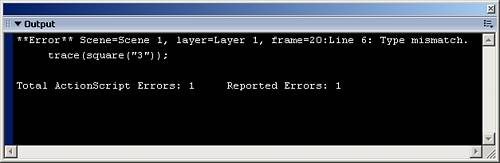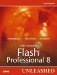# Strict Data Typing with Functions

A common problem in ActionScript 1.0 is building a function that is supposed to return a certain data type, a number for example, but, somehow it sends out a Boolean value instead. Or worse yet, you have parameters set to receive a certain data type, but another developer passes it a different data type, and then that developer cannot figure out where the error is. (More on functions in Chapter 12.)

Strict data typing in functions comes to the rescue. You cannot only data type the result of a function, but also all parameters in that function.

To data type the parameters of a function, you simply place a colon after each parameter name, and the data type pop-up list appears where you can choose which data type this parameter is meant to be.

To data type the return value of a function, you place a colon after the closing parenthesis of the parameters, and then choose which data type the return value is to be in.

Here is an example of data typing a function:

` //create the function function square(num:Number):Number{      return num*num; } //call the function trace(square(3)); //Output: 9 `

The preceding code creates the function with a single parameter, num. Both num and the result are set to the Number data type.

If the result does not match the strict data type, as in the following case, a similar error message will appear in the Output panel as in the earlier example.

` //create the function function square(num:Number):Number{      return "num*num"; } //call the function trace(square(3)); `

And what if the parameters placed in a calling function do not match the strict data types they were assigned, like this?

` //create the function function square(num:Number):Number{      return num*num; } //call the function trace(square("3")); `

This time the function is declared correctly, but when invoked, we use a string instead of a number.

Another error will appear, as shown in Figure 8.6.

##### Figure 8.6. Strict data typing can even make sure parameters match the correct data type declared.And because you can have strict data typing in both functions and variables, there is increased debugging help when combining them, as in the following example:

` //create the function function square(num:Number):Number{      return num*num; } //call the function var myName:String = square(3); `

This code creates the function we have been using, and this time sets the result to a variable that has a different data type from the one declared as the result returned from the function.

Run this code, and again you will receive another error message like Figure 8.6.

That concludes the discussion on the differences and features of ActionScript 2.0 for this chapter. Again, there is more on class construction in Chapter 18.

You have seen a lot of ActionScript already in this chapter, but what about all the features of the Actions panel?Macromedia Flash Professional 8 Unleashed
ISBN: 0672327619
EAN: 2147483647
Year: 2005
Pages: 319